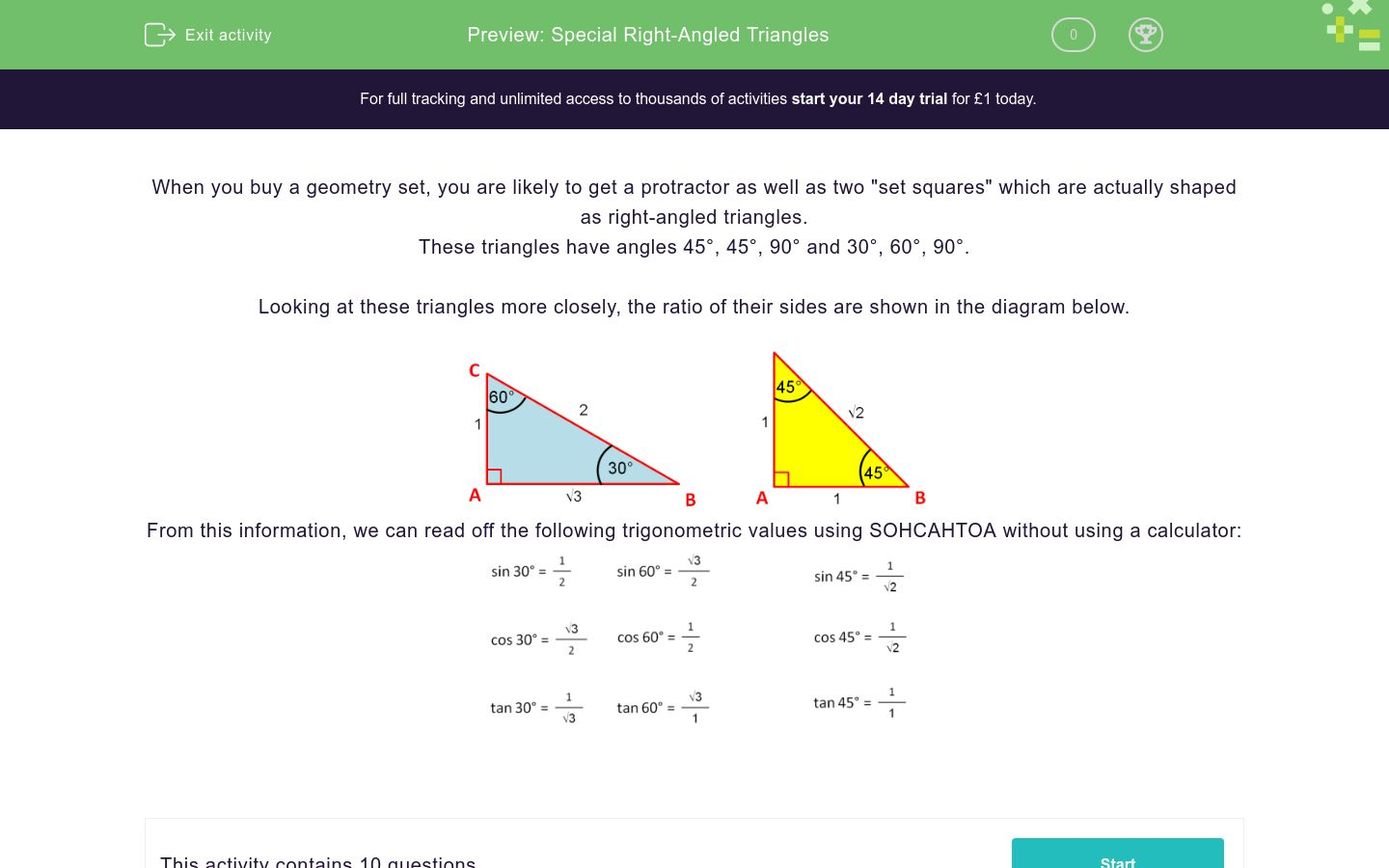# Special Right-Angled Triangles

In this worksheet, students answer trigonometric questions based on 45°, 45°, 90° and 30°, 60°, 90° triangles.Key stage:  KS 4

Curriculum topic:  Geometry and Measures

Difficulty level:### QUESTION 1 of 10

When you buy a geometry set, you are likely to get a protractor as well as two "set squares" which are actually shaped as right-angled triangles.

These triangles have angles 45°, 45°, 90° and 30°, 60°, 90°.

Looking at these triangles more closely, the ratio of their sides are shown in the diagram below.From this information, we can read off the following trigonometric values using SOHCAHTOA without using a calculator:Using one of the triangles shown below, state the value of:

tan 45°1

1/√2

1/√3

√3/2

2/√3

0.5

2

√3

√2

Using one of the triangles shown below, state the value of:

tan 30°1

1/√2

1/√3

√3/2

2/√3

0.5

2

√3

√2

Using one of the triangles shown below, state the value of:

sin 30°1

1/√2

1/√3

√3/2

2/√3

0.5

2

√3

√2

Using one of the triangles shown below, state the value of:

sin 45°1

1/√2

1/√3

√3/2

2/√3

0.5

2

√3

√2

Using one of the triangles shown below, state the value of:

cos 60°1

1/√2

1/√3

√3/2

2/√3

0.5

2

√3

√2

Using one of the triangles shown below, state the value of:

sin 60°1

1/√2

1/√3

√3/2

2/√3

0.5

2

√3

√2

Using one of the triangles shown below, state the value of:

cos 45°1

1/√2

1/√3

√3/2

2/√3

0.5

2

√3

√2

Using one of the triangles shown below, state the value of:

tan 60°1

1/√2

1/√3

√3/2

2/√3

0.5

2

√3

√2

Using one of the triangles shown below, state the value of:

cos 30°1

1/√2

1/√3

√3/2

2/√3

0.5

2

√3

√2

True or false?

cos 30° = sin 60°True

False

• Question 1

Using one of the triangles shown below, state the value of:

tan 45°CORRECT ANSWER
1
EDDIE SAYS
In the yellow triangle, tan 45° = opp/adj = 1/1
• Question 2

Using one of the triangles shown below, state the value of:

tan 30°CORRECT ANSWER
1/√3
EDDIE SAYS
In the blue triangle, tan 30° = opp/adj = 1/√3
• Question 3

Using one of the triangles shown below, state the value of:

sin 30°CORRECT ANSWER
0.5
EDDIE SAYS
In the blue triangle, sin 30° = opp/hyp = 1/2
• Question 4

Using one of the triangles shown below, state the value of:

sin 45°CORRECT ANSWER
1/√2
EDDIE SAYS
In the yellow triangle, sin 45° = opp/hyp = 1/√2
• Question 5

Using one of the triangles shown below, state the value of:

cos 60°CORRECT ANSWER
0.5
EDDIE SAYS
In the blue triangle, cos 60° = adj/hyp = 1/2
• Question 6

Using one of the triangles shown below, state the value of:

sin 60°CORRECT ANSWER
√3/2
EDDIE SAYS
In the blue triangle, sin 60° = opp/hyp = √3/2
• Question 7

Using one of the triangles shown below, state the value of:

cos 45°CORRECT ANSWER
1/√2
EDDIE SAYS
In the yellow triangle, cos 45° = adj/hyp = 1/√2
• Question 8

Using one of the triangles shown below, state the value of:

tan 60°CORRECT ANSWER
√3
EDDIE SAYS
In the blue triangle, tan 60° = opp/adj = √3/1
• Question 9

Using one of the triangles shown below, state the value of:

cos 30°CORRECT ANSWER
√3/2
EDDIE SAYS
In the blue triangle, cos 30° = adj/hyp = √3/2
• Question 10

True or false?

cos 30° = sin 60°CORRECT ANSWER
True
EDDIE SAYS
In the blue triangle,
cos 30° = adj/hyp = √3/2
sin 60° = opp/hyp = √3/2
---- OR ----

Sign up for a £1 trial so you can track and measure your child's progress on this activity.

### What is EdPlace?

We're your National Curriculum aligned online education content provider helping each child succeed in English, maths and science from year 1 to GCSE. With an EdPlace account you’ll be able to track and measure progress, helping each child achieve their best. We build confidence and attainment by personalising each child’s learning at a level that suits them.

Get startedStart your £1 trial today.
Subscribe from £10/month.